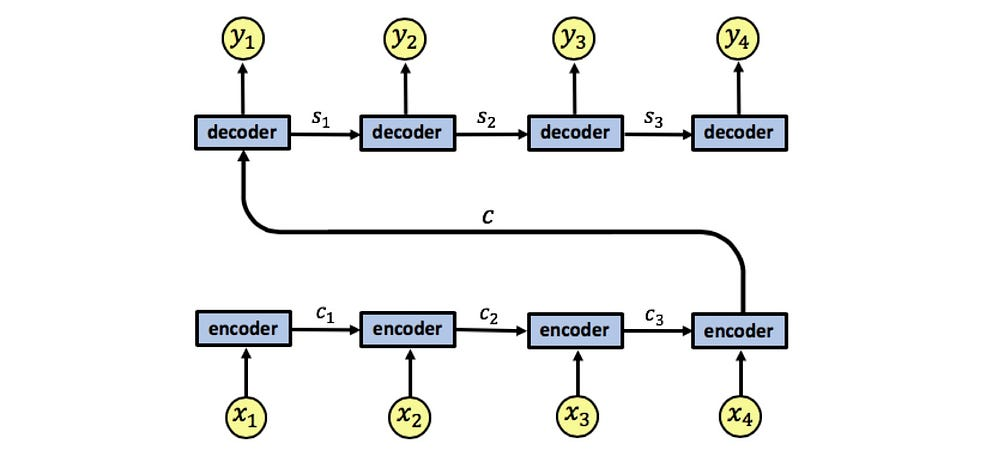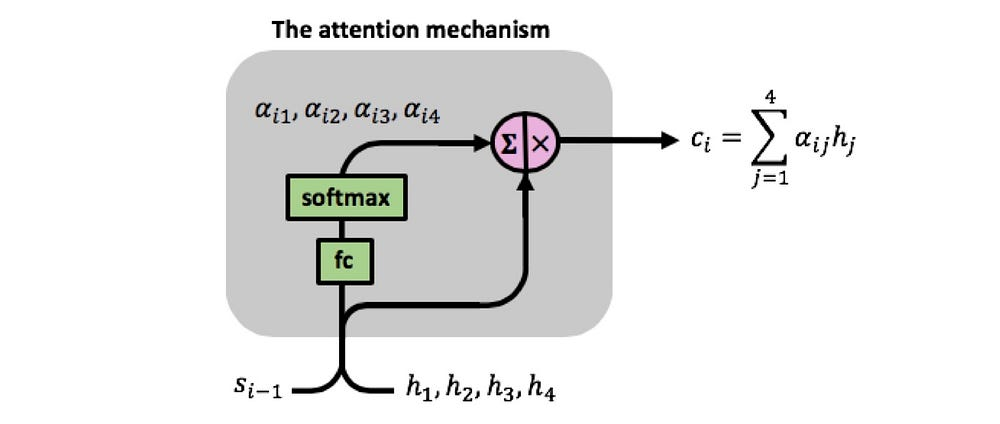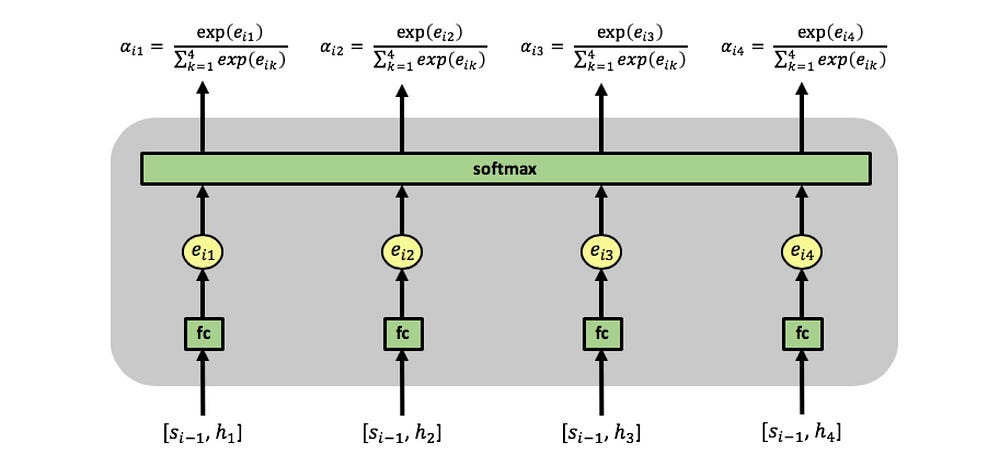# Attention in RNNs

Source: Deep Learning on Medium

## Understanding the mechanism with a detailed example

Recurrent Neural Networks (RNNs) have been used successfully for many tasks involving sequential data such as machine translation, sentiment analysis, image captioning, time-series prediction etc. Improved RNN models such as Long Short-Term Memory networks (LSTMs) enable training on long sequences overcoming problems like vanishing gradients. However, even the more advanced models have their limitations and researchers had a hard time developing high-quality models when working with long data sequences. In machine translation, for example, the RNN has to find connections between long input and output sentences composed of dozens of words. It seemed that the existing RNN architectures needed to be changed and adapted to better deal with such tasks.

Attention is a mechanism combined in the RNN allowing it to focus on certain parts of the input sequence when predicting a certain part of the output sequence, enabling easier learning and of higher quality. Combination of attention mechanisms enabled improved performance in many tasks making it an integral part of modern RNN networks.

This work is based on the paper Neural machine translation by jointly learning to align and translate by Bahdanau, Cho, and Bengio .

We start by briefly going over basic RNNs. The RNN encoder-decoder architecture we will focus on looks like this:An RNN encoder-decoder architecture, we take an architecture with 4 time steps for simplicity

The RNN encoder has an input sequence x1, x2, x3, x4. We denote the encoder states by c1, c2, c3. The encoder outputs a single output vector c which is passed as input to the decoder. Like the encoder, the decoder is also a single layered RNN, we denote the decoder states by s1, s2, s3 and the network’s output by y1, y2, y3, y4.

A problem with this architecture lies in the fact that the decoder needs to represent the entire input sequence x1, x2, x3, x4 as a single vector c, which can cause information loss. Moreover, the decoder needs to decipher the passed information from this single vector, a complex task in itself.

As detailed in :

A potential issue with this encoder–decoder approach is that a neural network needs to be able to compress all the necessary information of a source sentence into a fixed-length vector. This may make it difficult for the neural network to cope with long sentences, especially those that are longer than the sentences in the training corpus.

#### RNNs with an attention mechanism

An attention RNN looks like this:

Our attention model has a single layer RNN encoder, again with 4 time steps. We denote the encoder’s input vectors by x1, x2, x3, x4 and the output vectors by h1, h2, h3, h4.

The attention mechanism is located between the encoder and the decoder, its input is composed of the encoder’s output vectors h1, h2, h3, h4 and the states of the decoder s0, s1, s2, s3, the attention’s output is a sequence of vectors called context vectors denoted by c1, c2, c3, c4.

#### The context vectors

The context vectors enable the decoder to focus on certain parts of the input when predicting its output. Each context vector is a weighted sum of the encoder’s output vectors h1, h2, h3, h4, each vector hi contains information about the whole input sequence (since it has access to the encoder states during its computation) with a strong focus on the parts surrounding the i-th vector of the input sequence. The vectors h1, h2, h3, h4 are scaled by weights ɑij capturing the degree of relevance of input xj to output at time i, yi.

The context vectors c1, c2, c3, c4 are given by:

The attention weights are learned using an additional fully-connected shallow network, denoted by fc, this is where the s0, s1, s2, s3 part of the attention mechanism’s input comes into play. Computation of the attention weights is given by:

The attention weights are learned using the attention fully-connected network and a softmax function:At time step i, the mechanism has h1,h2,h3,h4 and si-1 as inputs, it uses the fc neural network and the softmax function to compute the attention weights αi1, αi2, αi3, αi4, these are then used in the computation of the context vector ci.

As can be seen in the above image, the fully-connected network receives the concatenation of vectors [si-1,hi] as input at time step i. The network has a single fully-connected layer, the outputs of the layer, denoted by eij, are passed through a softmax function computing the attention weights, which lie in [0,1].

Notice that we are using the same fully-connected network for all the concatenated pairs [s0,h1], [s1,h2], [s2,h3], [s3,h4], meaning there is a single network learning the attention weights.Computation of attention weights at time step i, notice how this needs to be computed separately on every time step since the computation at time step i involves si-1, the decoder’s state form time step i-1

The attention weights αij reflect the importance of hj with respect to the previous hidden state si−1 in deciding the next state si and generating yi. A large αij attention weight causes the RNN to focus on input xj (represented by the encoder’s output hj), when predicting the output yi.

The fc network is trained along with the encoder and decoder using backpropagation, the RNN’s prediction error terms are backpropagated backward through the decoder, then through the fc attention network and from there to the encoder.

Notice that since the attention weights are learned using an additional neural network fc, we have an additional set of weights allowing this learning to take place, we denote this weight matrix by Wa.

An RNN with 4 input time steps and 4 output time steps will have the following weight matrices fine-tuned during the training process. Note the dimensions 4×4 of the attention matrix, connecting between every input to every output:

This mechanism enables the decoder to decide which parts of the input sequence to pay attention to. By letting the decoder have an attention mechanism, we relieve the encoder from having to encode all information in the input sequence into a single vector. The information can be spread throughout the sequence h1, h2, h3, h4 which can be selectively retrieved by the decoder.

As written in :

The most important distinguishing feature of this approach from the basic encoder–decoder is that it does not attempt to encode a whole input sentence into a single fixed-length vector. Instead, it encodes the input sentence into a sequence of vectors and chooses a subset of these vectors adaptively while decoding the translation. This frees a neural translation model from having to squash all the information of a source sentence, regardless of its length, into a fixed-length vector. We show this allows a model to cope better with long sentences.

#### Computing the attention weights and context vectors

let’s go over a detailed example and see how the context vectors are computed.

The first act performed is the computation of vectors h1, h2, h3, h4 by the encoder. These are then used as inputs of the attention mechanism. This is where the decoder is first involved by inputting its initial state vector s0 and we have the first attention input sequence [s0, h1], [s0, h2], [s0, h3], [s0, h4].

The attention mechanism computes the first set of attention weights α11, α12, α13, α14 enabling the computation of the first context vector c1. The decoder now uses [s0,c1] and computes the first RNN output y1

At the following time step the attention mechanism has as input the sequence [s1, h1], [s1, h2], [s1, h3], [s1, h4].

It computes a second set of attention weights α21, α22, α23, α24 enabling the computation of the first context vector c2. The decoder uses [s1,c2] and computes the second RNN output y2.

At the next time step the attention mechanism has an input sequence [s2, h1], [s2, h2], [s2, h3], [s2, h4].

And computes a third set of attention weights α31, α32, α33, α34 enabling the computation of the third context vector c3. The decoder uses [s2,c3] and computes the following output y3.

At the next time step the attention mechanism has an input sequence [s3, h1], [s3, h2], [s3, h3], [s3, h4].

It computes a fourth set of attention weights α41, α42, α43, α44 enabling the computation of the fourth context vector c4. The decoder uses [s3,c4] and computes the final output y4.

We end with an example from , the task is English-French machine translation. Below are two alignments found by the attention RNN. The x-axis and y-axis of each plot correspond to the words in the source sentence (English) and the generated translation (French), respectively.

Each pixel shows the weight αij of the j-th source word and the i-th target word, in grayscale (0: black, 1: white).

We see how the attention mechanism allows the RNN to focus on a small part of the input sentence when outputting the translation. Notice how the larger attention parameters (given by the white pixels) connect corresponding parts of the English and French sentences enabling the network in  to achieve state of the art results.

References:  Neural Machine Translation By Jointly Learning To Align And Translate. Dzmitry Bahdanau, KyungHyun Cho and Yoshua Bengio.